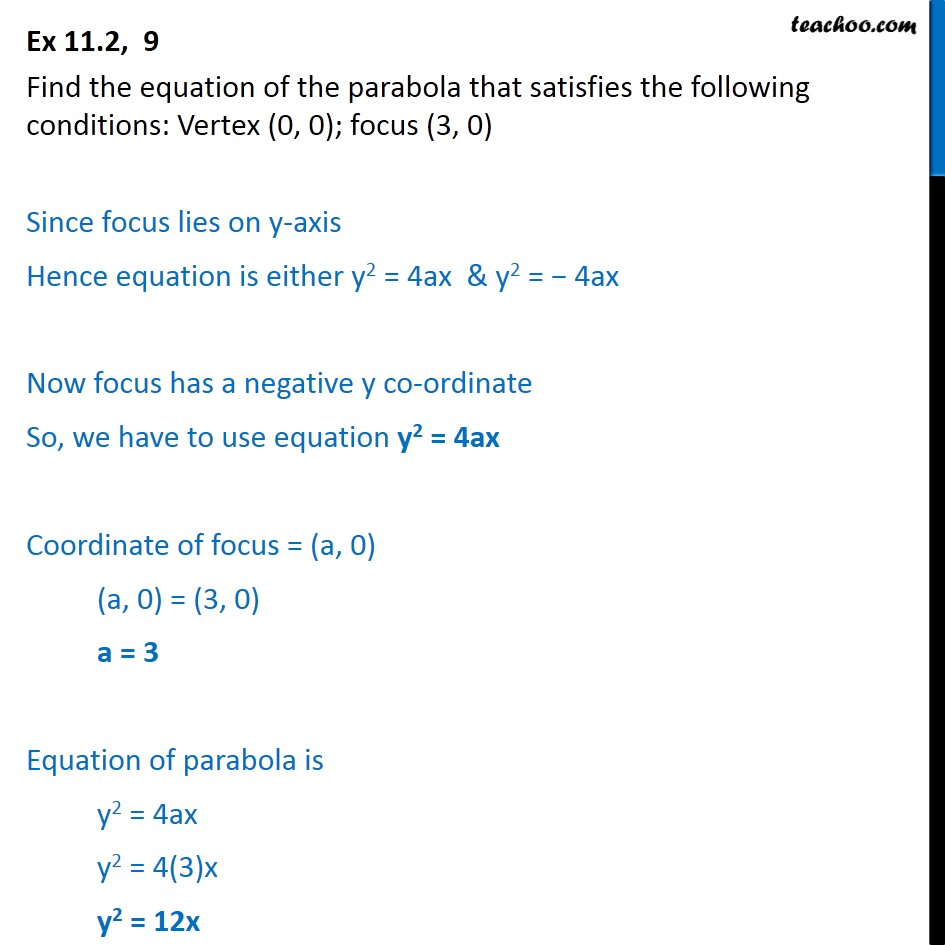Ex 10.2

Chapter 10 Class 11 Conic Sections
Serial order wiseLearn in your speed, with individual attention - Teachoo Maths 1-on-1 Class

### Transcript

Ex 10.2, 9 Find the equation of the parabola that satisfies the following conditions: Vertex (0, 0); focus (3, 0) Since focus lies on y-axis Hence equation is either y2 = 4ax & y2 = 4ax Now focus has a negative y co-ordinate So, we have to use equation y2 = 4ax Coordinate of focus = (a, 0) (a, 0) = (3, 0) a = 3 Equation of parabola is y2 = 4ax y2 = 4(3)x y2 = 12x